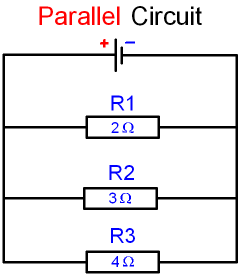gcsescience.com                                       24                                       gcsescience.com

Electricity

Parallel Circuits

What is the Resistance of a Parallel Circuit?

The total resistance (R total) of a
parallel circuit is calculated using the formula

1/R total = 1/R1 + 1/R2 + 1/R3 ·····In the above circuit,

1/R total = 1/2 + 1/3 + 1/4

= 6/12 + 4/12 + 3/12

1/R total = 13/12

R total  = 12/13

= 0·92 ohms.

Notice that this is a much smaller resistance
than a series circuit using the same resistors.
It is even smaller than the smallest resistor
in the parallel circuit above, which is 2 ohms.

Adding a resistor in an extra branch decreases
the total resistance of a parallel circuit
because the electricity has more paths to flow along
and so the total current flowing increases.

gcsescience.com         Physics Quiz         Index         Electricity Quiz         gcsescience.com